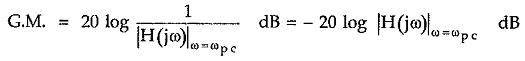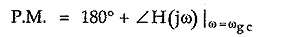## Specification from Bode Plot:

The important Specification from Bode Plot are,

1. Gain cross-over frequency (ωgc)
2. Phase cross-over frequency (ωpc)
3. Gain margin (G.M.)
4. Phase margin (P.M.)

### Gain Cross-over Frequency (ωgc):

The frequency at which the magnitude of system function is unity i.e. 0 dB is called gain cross over frequency. So frequency at which the resultant magnitude plot intersects 0 dB line is called gain cross over frequency denoted as ωgc.

### Phase Cross-over Frequency (ωpc):

The frequency at which the angle of the system function is -180° is called phase cross-over frequency denoted as ωpc.

### Gain Margin (G.M.):

As the system gain changes, the system stability gets affected. As gain K is increased, the system becomes less and less stable.

The gain margin is defined as the margin in gain allowable by which gain can be increased till the system reaches on the verge of instability.

The positive gain margin means increase in gain is allowable and system is stable. The negative gain margin means the gain limit has been crossed and system is unstable.

Mathematically it is defined as,In dB,More positive gain margin means more stable system.

### Phase Margin (P.M.):

Similar to the gain, the phase lag also can be introduced in the system which also affects the system stability.

The phase margin is defined as the amount of the additional phase lag which can be introduced in the system till system reaches on the verge of instability.

Mathematically it is defined as,More positive phase margin means more stable system

Scroll to Top